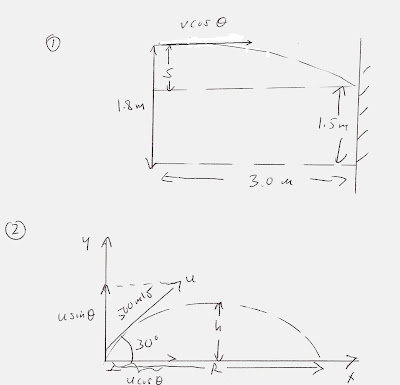## Wednesday, October 13, 2010

### Caleb Shay Scores 90% on Physics TestThough the basic physics test I posted earlier:

http://brane-space.blogspot.com/2010/10/basic-physics-test.html

was really intended for arrogant, presumptuous fundies to test their physics acumen (given how much they bloviate about "the 2nd law of thermodynamics" etc. in their religious jabber) I didn't exclude anyone from working them out. Turns out that Caleb Shay - who recently took down a certain egoistic pastor who dared to ask atheists impertinent questions, got 9 of 10 correct for a satisfying 90%. So I now post Caleb's answers in two parts, including the diagrams he sent by email (for the initial three problems).

#1 (See diagram (1))

a) The initial speed is referred to the horizontal component v cos (theta), so we have the displacement:

x = vt = (v cos(theta)) t

but v = v cos (theta) and since theta = 0 degrees, cos (0) = 1

So: v = x/t = 3.0 m/ t

where t is the time of flight

(b) By basic kinematics, the distance s the ball falls under gravity is:

s = 1/2 at^2 = gt^2/ 2

where g = 10 m/s^2

so time of flight t = [2s/ g] ^1/2 = [2 x (1.80m - 1.50m)/ 10 m/s^2]^1/2

t = [2(0.30m)/ 10 m/s^2]^1/2 = 0.245 s

so the answer to part (a) is:

v = 3.0 m/ 0.245s = 12.2 m/s

c) The speed when it strikes the board is the vector resultant of the horizontal component v(x) and the vertical v(y).

the horizontal is just the same as the initial speed, since v(x) = v cos(theta) = 12. 2 m/s

the vertical v(y) is the instantaneous 'drop' velocity (under g) so:

v(y) = ds/dt = gt = (10 m/s^2)(0.245s) = 2.45 m/s

so the resultant velocity is:

v = [v(x)^2 + v(y)^2]^1/2 = [(12.2 m/s)^2 + (2.45 m/s)^2]^1/2 = 12.44 m/s

(2)

(a) The horizontal range, R, see diagram is given by:

R = u^2 sin(2 theta)/ g = (500 m/s)^2 sin (60)/ 10 m/s^2

R = (250,000 m^2/s^2)(sin(60)/ 10 m/s^2 = 21, 650 m

(b) The greatest height, h can be obtained using:

v^2 = u^2 + 2 ah

From the sketch v = 0 at h (apex of flight) and a = (-g) therefore:

0 = (u sin(theta))^2 - 2 gh

so: h = (u^2 sin^2(30))/2g = (500 m/s)^2 (0.5)^2/ 2(10m/s^2)

h = 3 125 m

c) the time to reach the altitude h:

Since v = u + at

then: 0 = u (sin(theta)) - gt

t = u sin(theta)/ g = (500 m/s) sin (30)/ 10m/s^2 = 25 secs

(3) From the diagram:

we find the component of acceleration of the 2 kg mass along the incline is mg sin(theta)

This is also the component needed to apply Newton's 2nd law of motion: F = ma

(Force = mass times acceleration), so:

F = ma = mg sin(theta)

but theta = 30 degrees

so the acceleration: a = g sin (theta) = (10 m/s^2) sin (30) = 10 m/s^2(1/2)

or a = 2 m/s^2

(4) The a/c has a power of 1 kw, so to run it for five hours (11 a.m. to 4 p.m.) would require an energy total of: 1 kw x (5 h) = 5 kw-h

The Sun delivers 1360 watts per sq. meter, or 1.36 kw/ m^2

then the total collected by the collector area (5m x 5m = 25 m^2) is:

1.36 kw/ m^2 ( 25 m^2) = 34 kw

But it is only 12% efficient so that 88% of the power is wasted, and the useful part (per hour) is:

0.12 x (34 kw) = 4.08 kw

so that as long as the sun is out for the whole time, it will deliver:

(4.08 kw x 5h) = 20.4 kw-h

Since: 20.4 kw-h > 5.0 kw-h the panel can achieve its objective

(5) The heat lost is the same for both the alcohol (alc) and the water (H2O), so:

-Q = -Q(alc) = -Q(H2O)

Q(Al) = m(alc) c(alc) (dT)

and Q(H2O) = m(H2O) c(H2O) dT

where dT = (350 K - 300 K) = 50 K

m(alc) = 2 kg and m(H2O) = 1kg

c(H2O) = 4,200 J/kg K

and c(alc) is to be found

Then we can set up the heat equation:

m(alc) c(alc) (dT) = m(H2O) c(H2O) dT

and solve for:

c(alc) = [m(H2O) c(H2O) ] / m(alc)

where the dT cancels on each side

c(alc) = [1 kg ( 4,200 J/kg K)]/ 2 kg = 2, 100 J/ Kg K

----

To be continued...including looking at Caleb's wrong answer!